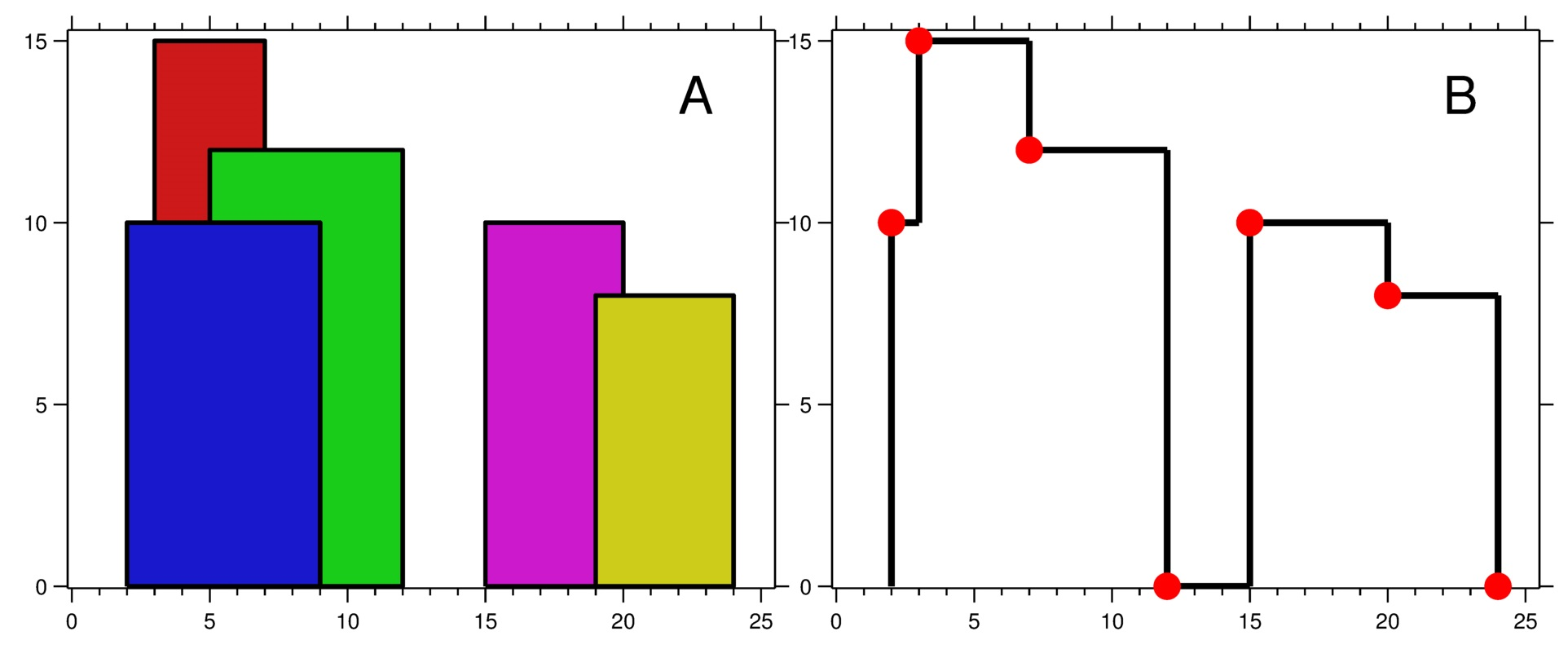218. The Skyline Problem

A city's skyline is the outer contour of the silhouette formed by all the buildings in that city when viewed from a distance. Given the locations and heights of all the buildings, return the skyline formed by these buildings collectively.

The geometric information of each building is given in the array `buildings` where `buildings[i] = [lefti, righti, heighti]`:

• `lefti` is the x coordinate of the left edge of the `ith` building.
• `righti` is the x coordinate of the right edge of the `ith` building.
• `heighti` is the height of the `ith` building.

You may assume all buildings are perfect rectangles grounded on an absolutely flat surface at height `0`.

The skyline should be represented as a list of "key points" sorted by their x-coordinate in the form `[[x1,y1],[x2,y2],...]`. Each key point is the left endpoint of some horizontal segment in the skyline except the last point in the list, which always has a y-coordinate `0` and is used to mark the skyline's termination where the rightmost building ends. Any ground between the leftmost and rightmost buildings should be part of the skyline's contour.

Note: There must be no consecutive horizontal lines of equal height in the output skyline. For instance, `[...,[2 3],[4 5],[7 5],[11 5],[12 7],...]` is not acceptable; the three lines of height 5 should be merged into one in the final output as such: `[...,[2 3],[4 5],[12 7],...]`

Example 1:```Input: buildings = [[2,9,10],[3,7,15],[5,12,12],[15,20,10],[19,24,8]]
Output: [[2,10],[3,15],[7,12],[12,0],[15,10],[20,8],[24,0]]
Explanation:
Figure A shows the buildings of the input.
Figure B shows the skyline formed by those buildings. The red points in figure B represent the key points in the output list.
```

Example 2:

```Input: buildings = [[0,2,3],[2,5,3]]
Output: [[0,3],[5,0]]
```

Constraints:

• `1 <= buildings.length <= 104`
• `0 <= lefti < righti <= 231 - 1`
• `1 <= heighti <= 231 - 1`
• `buildings` is sorted by `lefti` in non-decreasing order.

218. The Skyline Problem
``````struct Solution;

use std::cmp::Ordering::*;
use std::collections::VecDeque;

impl Solution {
fn get_skyline(buildings: Vec<Vec<i32>>) -> Vec<Vec<i32>> {
if buildings.is_empty() {
return vec![];
}
let mut queue: VecDeque<Vec<Vec<i32>>> = buildings
.into_iter()
.map(|x| vec![vec![x, x], vec![x, 0]])
.collect();
while queue.len() > 1 {
let a = queue.pop_front().unwrap();
let b = queue.pop_front().unwrap();
let c = Self::merge(a, b);
queue.push_back(c);
}
queue.pop_front().unwrap()
}

fn merge(a: Vec<Vec<i32>>, b: Vec<Vec<i32>>) -> Vec<Vec<i32>> {
let mut i = 0;
let mut j = 0;
let mut res = vec![];
let mut prev_h = 0;
let mut l = 0;
let mut r = 0;
let mut x;
while i < a.len() && j < b.len() {
match a[i].cmp(&b[j]) {
Equal => {
x = a[i];
l = a[i];
r = b[j];
i += 1;
j += 1;
}
Less => {
x = a[i];
l = a[i];
i += 1;
}
Greater => {
x = b[j];
r = b[j];
j += 1;
}
}
let h = l.max(r);
if h != prev_h {
res.push(vec![x, h]);
prev_h = h;
}
}
while i < a.len() {
let x = a[i];
let h = a[i];
i += 1;
if h != prev_h {
res.push(vec![x, h]);
prev_h = h;
}
}
while j < b.len() {
let x = b[j];
let h = b[j];
j += 1;
if h != prev_h {
res.push(vec![x, h]);
prev_h = h;
}
}
res
}
}

#[test]
fn test() {
let buildings = vec_vec_i32![
[2, 9, 10],
[3, 7, 15],
[5, 12, 12],
[15, 20, 10],
[19, 24, 8]
];
let res = vec![
[2, 10],
[3, 15],
[7, 12],
[12, 0],
[15, 10],
[20, 8],
[24, 0],
];
assert_eq!(Solution::get_skyline(buildings), res);
}
``````